``````knitr::opts_chunk\$set(echo = TRUE, cache = FALSE, eval = TRUE,
warning = TRUE, message = TRUE)``````

## Introduction

Although multidimensional single-cell-based flow and mass cytometry have been increasingly applied to microenvironmental composition and stem-cell research, integrated analysis workflows to facilitate the interpretation of experimental cytometry data remain underdeveloped. We present flowSpy, a comprehensive R package designed for the analysis and interpretation of flow and mass cytometry data. We applied flowSpy to mass cytometry and time-course flow cytometry data to demonstrate the usage and practical utility of its computational modules. flowSpy is a reliable tool for multidimensional cytometry data workflows and produces compelling results for trajectory construction and pseudotime estimation.

## Overview of flowSpy workflow

The flowSpy package is developed to complete the majority of standard analysis and visualization workflow for FCS data. In flowSpy workflow, an S4 object in R is built to implement the statistical and computational approach, and all computational modules are integrated into one single channel which only requires a specified input data format. Computational modules of flowSpy can be divided into four main parts (Fig. 1): preprocessing, trajectory, analysis and visualization.

• Preprocessing. Data import, compensation, quality control, filtration, normalization and merge cells from different samples can be implemented in the preprocessing module. After preprocessing, a matrix contains clean cytometric signaling data, a data.frame containing meta-information of the experiment and a vector contains all markers enrolled in the computational process are required to build an FPSY object.

• Trajectory. Cells built in the FSPY object are classfied into different clusters based on the expression level of input markers. You can choose different clustering methods by inputting different parameters. After clustering, cells are downsampled in a cluster-dependent fashion to reduce the total cell size and avoid small cluster deletion. Dimensionality reduction for both cells and clusters are also implemented in the clustering procedure. After dimensionality reduction, we use Minimus Spanning Tree (MST) to construct cell trajectory.

• Analysis. This module is design for time couse FCS data. Before running pseudotime, root cells must be defined first based on users’ priori knowledge. Root cells in flowSpy workflow are the initial cells of the trajectory tree. So it can be set using one vertex node of the tree or a cluster of cells with specific antibodies combination. Intermediate state evaluation is also envolved in the pseudotime module. Leaf cells are defined by the end node of trajectory tree or the end stage of the experiment. Intermediate state cells are cells with higher betweenness in the graph built on cell-cell connection, which play an important role between the connection of root cells and leaf cells.

• Visualization. The visualization module can provide clear and concise visualization of FCS data in an effective and easy-to-comprehend manner. flowSpy package offers various plotting functions to generate customizable and publication-quality plots. Two-dimensional or three-dimensional plot can fit most requirement from dimensionality reduction results. And tree-based plot can visualize cell trajectory as force-directed layout tree. Other special plots such as heatmap and violin plot are also provided in flowSpy.

``````# Loading packages
suppressMessages({
library(ggplot2)
library(flowSpy)
library(flowCore)
library(stringr)
})

fcs.path <- system.file("extdata", package = "flowSpy")
fcs.files <- list.files(fcs.path, pattern = '.FCS\$', full = TRUE)

fcs.data <- runExprsMerge(fcs.files, comp = FALSE, transformMethod = "none")

# Refine colnames of fcs data
recol <- c(`FITC-A<CD43>` = "CD43", `APC-A<CD34>` = "CD34",
`BV421-A<CD90>` = "CD90", `BV510-A<CD45RA>` = "CD45RA",
`BV605-A<CD31>` = "CD31", `BV650-A<CD49f>` = "CD49f",
`BV 735-A<CD73>` = "CD73", `BV786-A<CD45>` = "CD45",
`PE-A<FLK1>` = "FLK1", `PE-Cy7-A<CD38>` = "CD38")
colnames(fcs.data)[match(names(recol), colnames(fcs.data))] = recol
fcs.data <- fcs.data[, recol]

day.list <- c("D0", "D2", "D4", "D6", "D8", "D10")
meta.data <- data.frame(cell = rownames(fcs.data),
stage = str_replace(rownames(fcs.data), regex(".FCS.+"), "") )
meta.data\$stage <- factor(as.character(meta.data\$stage), levels = day.list)

markers <- c("CD43","CD34","CD90","CD45RA","CD31","CD49f","CD73","CD45","FLK1","CD38")

# Build the FSPY object
fspy <- createFSPY(raw.data = fcs.data, markers = markers,
meta.data = meta.data,
normalization.method = "log",
verbose = TRUE)``````
``## 2020-04-20 15:31:10 [INFO] Number of cells in processing: 600``
``## 2020-04-20 15:31:10 [INFO] rownames of meta.data and raw.data will be named using column cell``
``## 2020-04-20 15:31:10 [INFO] Index of markers in processing``
``## 2020-04-20 15:31:10 [INFO] Creating FSPY object.``
``## 2020-04-20 15:31:10 [INFO] Determining normalization factors``
``## 2020-04-20 15:31:10 [INFO] Normalization and log-transformation.``
``## 2020-04-20 15:31:10 [INFO] Build FSPY object succeed``
``````# See information
fspy``````
``````## FSPY Information:
##  Input cell number: 600  cells
##  Enroll marker number: 10  markers
##  Cells after downsampling: 600  markers``````
``````# Cluster cells by SOM algorithm
# Set random seed to make results reproducible
set.seed(1)
fspy <- runCluster(fspy, cluster.method = "som")``````
``## Mapping data to SOM``
``````# Do not perform downsampling
set.seed(1)
fspy <- processingCluster(fspy)

# run Principal Component Analysis (PCA)
fspy <- runFastPCA(fspy)

# run t-Distributed Stochastic Neighbor Embedding (tSNE)
fspy <- runTSNE(fspy)

# run Diffusion map
fspy <- runDiffusionMap(fspy)

# run Uniform Manifold Approximation and Projection (UMAP)
fspy <- runUMAP(fspy)

# build minimum spanning tree based on tsne
fspy <- buildTree(fspy, dim.type = "tsne", dim.use = 1:2)

# DEGs of different branch
diff.list <- runDiff(fspy)

# define root cells
fspy <- defRootCells(fspy, root.cells = c(28,26))

# run pseudotime
fspy <- runPseudotime(fspy, verbose = TRUE, dim.type = "raw")``````
``## 2020-04-20 15:31:16 [INFO] Calculating Pseudotime.``
``## 2020-04-20 15:31:16 [INFO] Pseudotime exists in meta.data, it will be replaced.``
``## 2020-04-20 15:31:16 [INFO] The log data will be used to calculate trajectory``
``## 2020-04-20 15:31:17 [INFO] Calculating Pseudotime completed.``
``````# define leaf cells
fspy <- defLeafCells(fspy, leaf.cells = c(27, 13), verbose = TRUE)``````
``## 2020-04-20 15:31:17 [INFO] 37 cells will be added to leaf.cells .``
``````# run walk between root cells and leaf cells
fspy <- runWalk(fspy, verbose = TRUE)``````
``## 2020-04-20 15:31:17 [INFO] Calculating walk between root.cells and leaf.cells .``
``## 2020-04-20 15:31:17 [INFO] Generating an adjacency matrix.``
``## 2020-04-20 15:31:17 [INFO] Walk forward.``
``## 2020-04-20 15:31:17 [INFO] Calculating walk completed.``
``````# Save object
if (FALSE) {
save(fspy, file = "Path to you output directory")
}

######################## Visualization

# Plot 2D tSNE. And cells are colored by cluster id
plot2D(fspy, item.use = c("tSNE_1", "tSNE_2"), color.by = "cluster.id",
alpha = 1, main = "tSNE", category = "categorical", show.cluser.id = TRUE)``````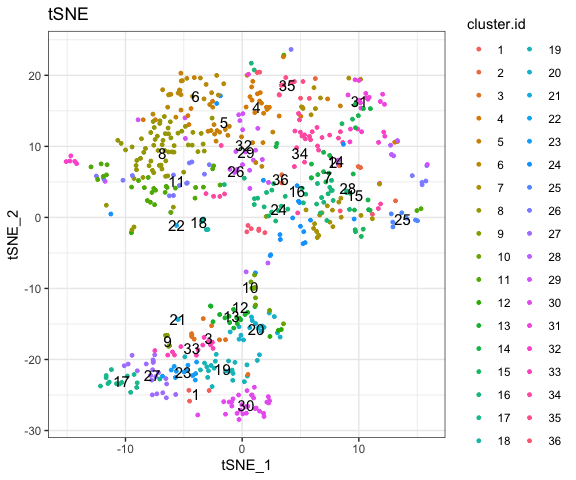``````# Plot 2D UMAP. And cells are colored by cluster id
plot2D(fspy, item.use = c("UMAP_1", "UMAP_2"), color.by = "cluster.id",
alpha = 1, main = "UMAP", category = "categorical", show.cluser.id = TRUE)``````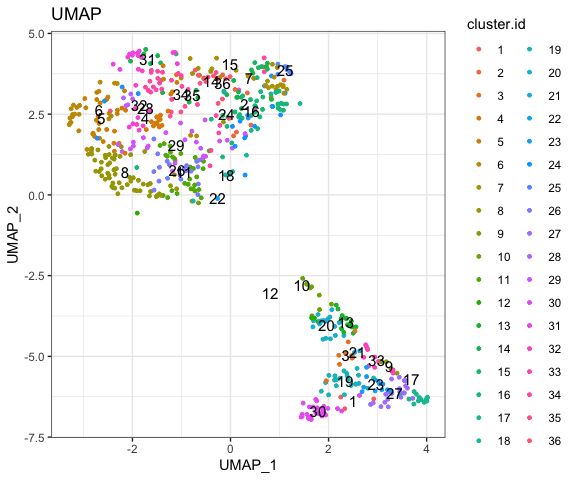``````# Plot 2D tSNE. And cells are colored by cluster id
plot2D(fspy, item.use = c("tSNE_1", "tSNE_2"), color.by = "branch.id",
alpha = 1, main = "tSNE", category = "categorical", show.cluser.id = TRUE)``````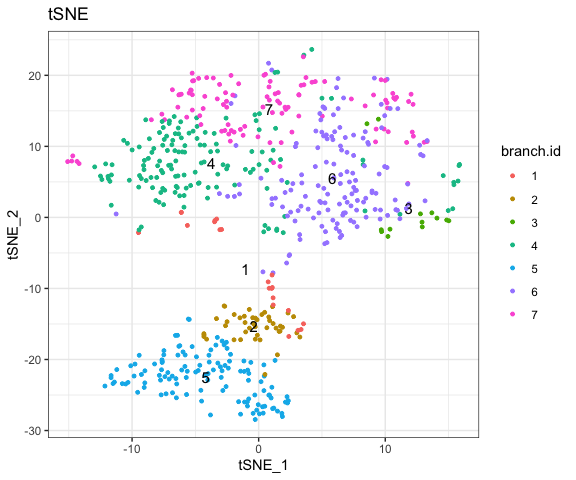``````# Plot 2D UMAP. And cells are colored by cluster id
plot2D(fspy, item.use = c("UMAP_1", "UMAP_2"), color.by = "branch.id",
alpha = 1, main = "UMAP", category = "categorical", show.cluser.id = TRUE)``````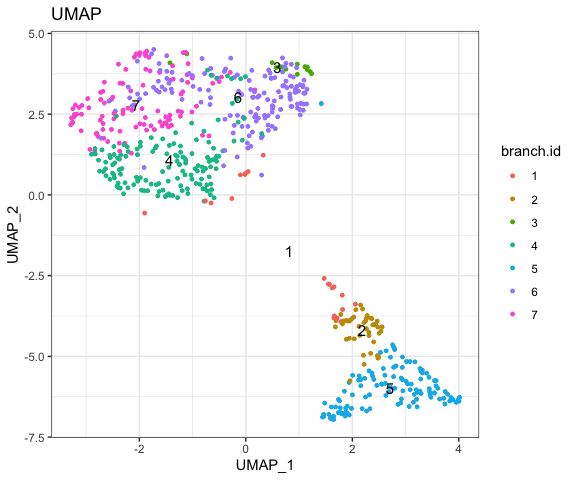``````# Plot 2D tSNE. And cells are colored by stage
plot2D(fspy, item.use = c("tSNE_1", "tSNE_2"), color.by = "stage",
alpha = 1, main = "UMAP", category = "categorical") +
scale_color_manual(values = c("#00599F","#009900","#FF9933",
"#FF99FF","#7A06A0","#FF3222"))``````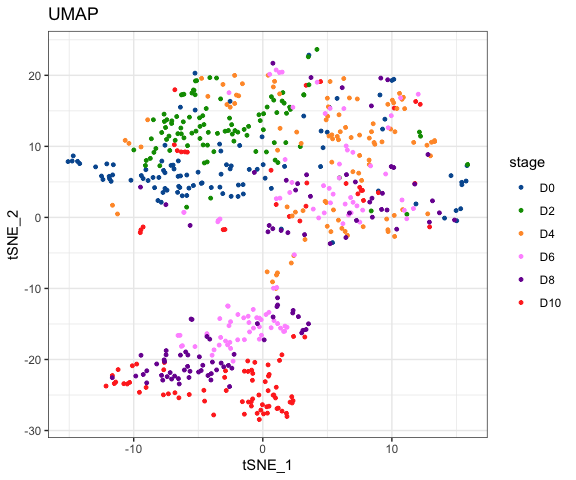``````# Plot 2D UMAP. And cells are colored by stage
plot2D(fspy, item.use = c("UMAP_1", "UMAP_2"), color.by = "stage",
alpha = 1, main = "UMAP", category = "categorical") +
scale_color_manual(values = c("#00599F","#009900","#FF9933",
"#FF99FF","#7A06A0","#FF3222"))``````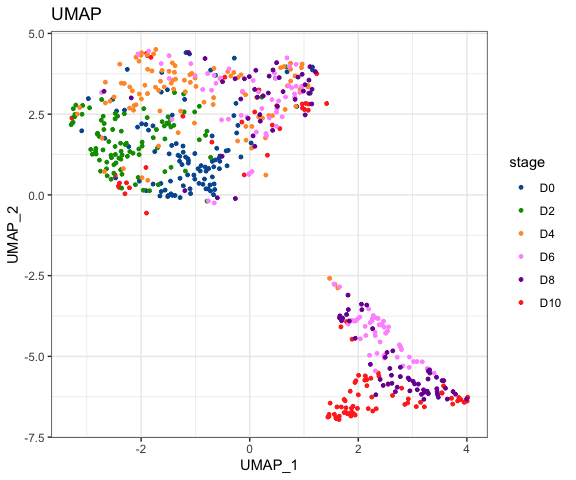``````# Tree plot
plotTree(fspy, color.by = "D0.percent", show.node.name = TRUE, cex.size = 1) +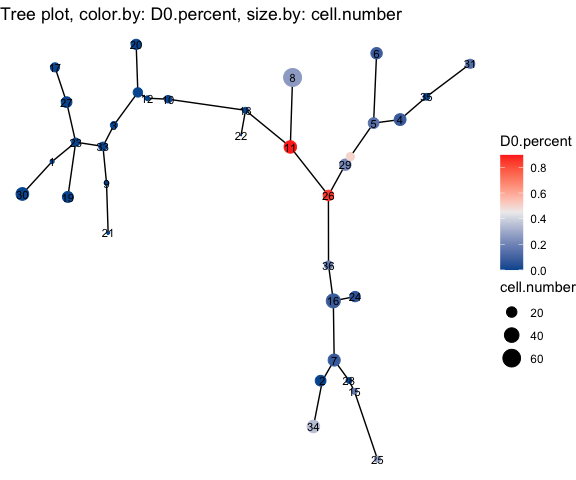``````plotTree(fspy, color.by = "CD43", show.node.name = TRUE, cex.size = 1) +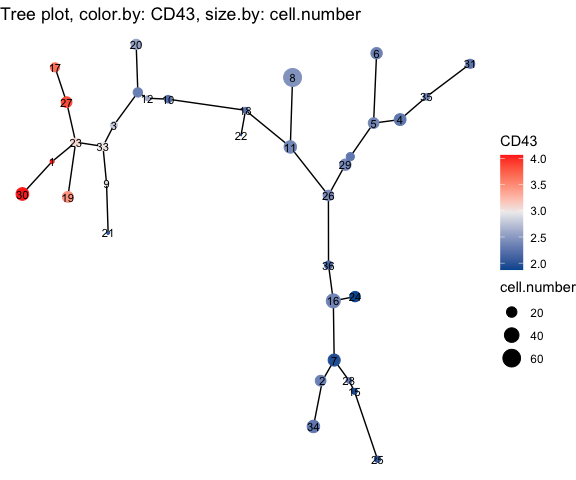``````# plot clusters
plotCluster(fspy, item.use = c("tSNE_1", "tSNE_2"), category = "numeric",
size = 100, color.by = "CD45RA") +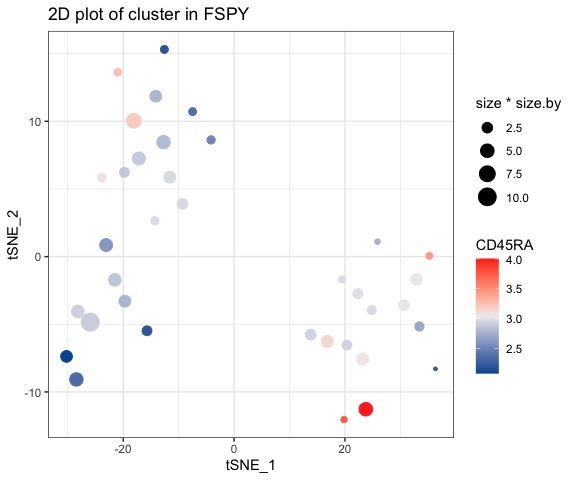``````# plot pie tree
plotPieTree(fspy, cex.size = 3, size.by.cell.number = TRUE) +
scale_fill_manual(values = c("#00599F","#FF3222","#009900",
"#FF9933","#FF99FF","#7A06A0"))``````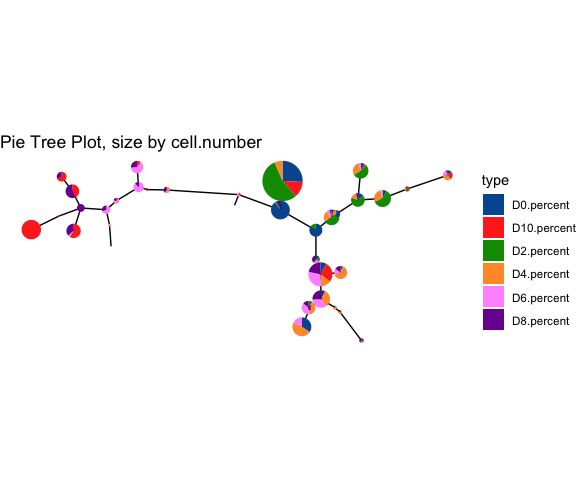``````# plot pie cluster
plotPieCluster(fspy, item.use = c("tSNE_1", "tSNE_2"), cex.size = 40) +
scale_fill_manual(values = c("#00599F","#FF3222","#009900",
"#FF9933","#FF99FF","#7A06A0"))``````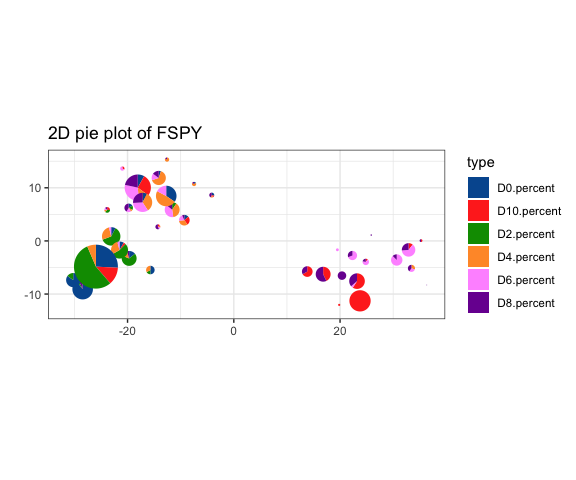``````# plot heatmap of cluster
plotClusterHeatmap(fspy)``````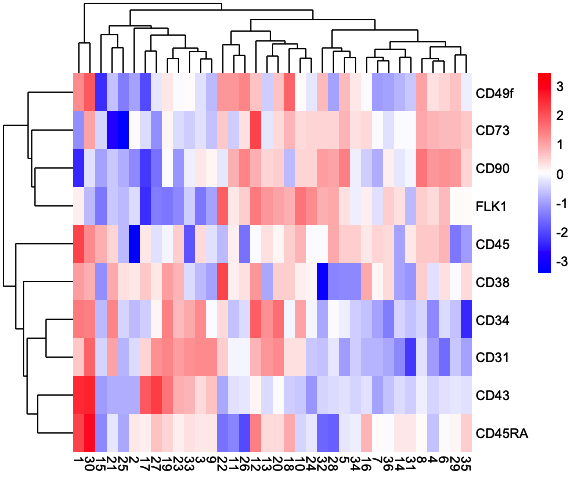``plotBranchHeatmap(fspy)``